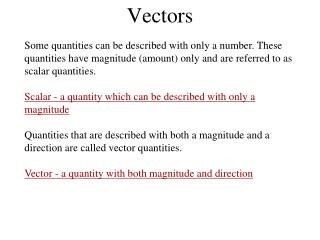# Vectors - PowerPoint PPT PresentationDownload PresentationVectors

VectorsDownload Presentation## Vectors

- - - - - - - - - - - - - - - - - - - - - - - - - - - E N D - - - - - - - - - - - - - - - - - - - - - - - - - - -
##### Presentation Transcript

1. Vectors Some quantities can be described with only a number. These quantities have magnitude (amount) only and are referred to as scalar quantities. Scalar - a quantity which can be described with only a magnitude Quantities that are described with both a magnitude and a direction are called vector quantities. Vector - a quantity with both magnitude and direction

2. Vectors We can use a graphical method to help us picture vectors, and to allow us to understand how vectors are added and subtracted. We will represent vector quantities with arrows. The length of the arrow represents its magnitude, the arrow tip and the direction of the arrow represent the vector direction. tip tail The point of the arrow is referred to as the “Tip”. The non-pointed end is called the “Tail”

3. The length and direction gives all the info about a vector. Vectors A and B are completely equivalent. B = 12 m east A = 12 m east A = B Vectors A vector can be moved (translated) without changing it. As long as the length and direction do not change, the vector has not changed.

4. If vectors are to be worked with graphically, axes and an appropriate scale must be chosen. Suppose we are describing the motion of a bug walking on the ground. If the bug walks 12 m to the east, we call this the bug’s displacement. We would write this as A = 12 m east . Once we establish a coordinate system, we can represent this displacement vector with an arrow like the one below. N W E S Vectors A = 12 m east

5. Vector Addition The process of adding two or more vectors is called Vector Addition. The sum of two or more vectors is called the resultant vector.

6. N C B W E A S C = A + B Vector Addition “Triangle Method” of Vector Addition Steps for adding two vectors using the tip-to-tail method: 1) Draw the first vector (A) to scale, with its tail at the origin 2) Draw the second vector (B) also to scale, with its tail at the tip of the first vector 3) The resultant vector runs from the tail end of the first vector to the tip of the second vector.

7. N C B W E A S C = A + B “Parallelogram Method” of Vector Addition Vector Addition Steps for adding two vectors using the parallelogram method: 1) Draw the first vector (A) to scale, with its tail at the origin 2) Draw the second vector (B) also to scale, and also with its tail at the origin. 3) Starting at the tip of one vector, draw a dotted line parallel to the other vector. Repeat, starting from the tip of the second vector. 4) The resultant vector runs from the origin to the intersection of the two dotted lines.

8. Notice - whichever method you use, you get the same value for vector C ! N N C C B B W E W E A A S S C = A + B C = A + B Tip - to - Tail Method Parallelogram Method Vector Addition

9. N N B B W W E E C C A A S S A + B = C A + B = C Vector Addition The methods work no matter the direction of the original vectors. Tip - to - Tail Method Parallelogram Method

10. N N C D D B B W W E E C A A S S C + B + A = D A + B + C = D Vector Addition If you need to add more than two vectors, the tip-to-tail method is usually easiest. Note that the order the vectors are added does not affect the result.

11. A -A Vector Subtraction For any vector A, the vector -A is simply a vector equal in magnitude and opposite in direction to A.

12. Subtracting one vector from another is the same as adding a negative vector. A - B = A + (-B) addition: A + B A + B = C C B B -B -B A A A A subtraction: A - B A + (-B) = D D Vector Subtraction

13. N  C B W E A S C = A + B Tip - to - Tail Method Vector addition: Mathematical Method To add two perpendicular vectors mathematically, make a sketch of the two vectors you are adding, and use either graphical method to sketch their resultant. To find the magnitude of the resultant, use the Pythagorean theorem. To find the direction of the resultant, use an inverse trig function, for example: tan-1 Tan = B/A = tan-1(B/A)

14. N CN C W E CE S Finding Vector Components: Mathematical Method To break a vector down into perpendicular components, first make a sketch of the vector. Sketch dotted lines from the tip of the vector and parallel to each axis. Draw in the components, from the origin to the dotted line.  To calculate the magnitude of the components, use the sin and cos functions. Sin = CN/C C = CE + CN CN = C sin CE = C cos Cos = CE/C